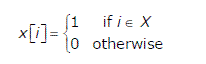# GATE | GATE-CS-2006 | Question 50

A set X can be represented by an array x[n] as follows:Consider the following algorithm in which x,y and z are Boolean arrays of size n:

 `algorithm zzz(x[] , y[], z []) ` `{ ` `   ``int` `i; ` `   ``for` `(i=O; i

The set Z computed by the algorithm is:
(A) (X ∩ Y)
(B) (X ∪ Y)
(C) (X-Y) ∩ (Y-X)
(D) (X-Y) ∪ (Y-X)

Explanation: Same as http://quiz.geeksforgeeks.org/algorithms-misc-question-4/

Quiz of this Question

My Personal Notes arrow_drop_up

Article Tags :

Be the First to upvote.

Please write to us at contribute@geeksforgeeks.org to report any issue with the above content.